Elementary Algebra

# 10.4Solve Applications Modeled by Quadratic Equations

Elementary Algebra10.4 Solve Applications Modeled by Quadratic Equations

### Learning Objectives

By the end of this section, you will be able to:

• Solve applications modeled by Quadratic Equations

### Be Prepared 10.4

Before you get started, take this readiness quiz.

1. The sum of two consecutive odd numbers is $−100−100$. Find the numbers.
If you missed this problem, review Example 3.10.
2. The area of triangular mural is 64 square feet. The base is 16 feet. Find the height.
If you missed this problem, review Example 3.36.
3. Find the length of the hypotenuse of a right triangle with legs 5 inches and 12 inches.
If you missed this problem, review Example 3.39.

### Solve Applications of the Quadratic Formula

We solved some applications that are modeled by quadratic equations earlier, when the only method we had to solve them was factoring. Now that we have more methods to solve quadratic equations, we will take another look at applications. To get us started, we will copy our usual Problem Solving Strategy here so we can follow the steps.

### How To

#### Use the problem solving strategy.

1. Step 1. Read the problem. Make sure all the words and ideas are understood.
2. Step 2. Identify what we are looking for.
3. Step 3. Name what we are looking for. Choose a variable to represent that quantity.
4. Step 4. Translate into an equation. It may be helpful to restate the problem in one sentence with all the important information. Then, translate the English sentence into an algebra equation.
5. Step 5. Solve the equation using good algebra techniques.
6. Step 6. Check the answer in the problem and make sure it makes sense.
7. Step 7. Answer the question with a complete sentence.

We have solved number applications that involved consecutive even integers and consecutive odd integers by modeling the situation with linear equations. Remember, we noticed each even integer is 2 more than the number preceding it. If we call the first one n, then the next one is $n+2n+2$. The next one would be $n+2+2n+2+2$ or $n+4n+4$. This is also true when we use odd integers. One set of even integers and one set of odd integers are shown below.

$Consecutive even integersConsecutive odd integers64,66,6877,79,81n1steven integern+22ndconsecutive even integern+43rdconsecutive even integern1stodd integern+22ndconsecutive odd integern+43rdconsecutive odd integerConsecutive even integersConsecutive odd integers64,66,6877,79,81n1steven integern+22ndconsecutive even integern+43rdconsecutive even integern1stodd integern+22ndconsecutive odd integern+43rdconsecutive odd integer$

Some applications of consecutive odd integers or consecutive even integers are modeled by quadratic equations. The notation above will be helpful as you name the variables.

### Example 10.38

The product of two consecutive odd integers is 195. Find the integers.

### Try It 10.75

The product of two consecutive odd integers is 99. Find the integers.

### Try It 10.76

The product of two consecutive odd integers is 168. Find the integers.

We will use the formula for the area of a triangle to solve the next example.

### Area of a Triangle

For a triangle with base $bb$ and height $hh$, the area, $AA$, is given by the formula $A=12bhA=12bh$.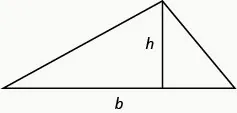Recall that, when we solve geometry applications, it is helpful to draw the figure.

### Example 10.39

An architect is designing the entryway of a restaurant. She wants to put a triangular window above the doorway. Due to energy restrictions, the window can have an area of 120 square feet and the architect wants the width to be 4 feet more than twice the height. Find the height and width of the window.

### Try It 10.77

Find the dimensions of a triangle whose width is four more than six times its height and has an area of 208 square inches.

### Try It 10.78

If a triangle that has an area of 110 square feet has a height that is two feet less than twice the width, what are its dimensions?

In the two preceding examples, the number in the radical in the Quadratic Formula was a perfect square and so the solutions were rational numbers. If we get an irrational number as a solution to an application problem, we will use a calculator to get an approximate value.

The Pythagorean Theorem gives the relation between the legs and hypotenuse of a right triangle. We will use the Pythagorean Theorem to solve the next example.

### Pythagorean Theorem

In any right triangle, where $aa$ and $bb$ are the lengths of the legs and $cc$ is the length of the hypotenuse, $a2+b2=c2a2+b2=c2$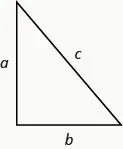### Example 10.40

Rene is setting up a holiday light display. He wants to make a ‘tree’ in the shape of two right triangles, as shown below, and has two 10-foot strings of lights to use for the sides. He will attach the lights to the top of a pole and to two stakes on the ground. He wants the height of the pole to be the same as the distance from the base of the pole to each stake. How tall should the pole be?

### Try It 10.79

The sun casts a shadow from a flag pole. The height of the flag pole is three times the length of its shadow. The distance between the end of the shadow and the top of the flag pole is 20 feet. Find the length of the shadow and the length of the flag pole. Round to the nearest tenth of a foot.

### Try It 10.80

The distance between opposite corners of a rectangular field is four more than the width of the field. The length of the field is twice its width. Find the distance between the opposite corners. Round to the nearest tenth.

### Example 10.41

Mike wants to put 150 square feet of artificial turf in his front yard. This is the maximum area of artificial turf allowed by his homeowners association. He wants to have a rectangular area of turf with length one foot less than three times the width. Find the length and width. Round to the nearest tenth of a foot.

### Try It 10.81

The length of a 200 square foot rectangular vegetable garden is four feet less than twice the width. Find the length and width of the garden. Round to the nearest tenth of a foot.

### Try It 10.82

A rectangular tablecloth has an area of 80 square feet. The width is 5 feet shorter than the length. What are the length and width of the tablecloth? Round to the nearest tenth of a foot.

The height of a projectile shot upwards is modeled by a quadratic equation. The initial velocity, $v0v0$, propels the object up until gravity causes the object to fall back down.

### Projectile Motion

The height in feet, $hh$, of an object shot upwards into the air with initial velocity, $v0v0$, after $tt$ seconds is given by the formula:

$h=−16t2+v0th=−16t2+v0t$

We can use the formula for projectile motion to find how many seconds it will take for a firework to reach a specific height.

### Example 10.42

A firework is shot upwards with initial velocity 130 feet per second. How many seconds will it take to reach a height of 260 feet? Round to the nearest tenth of a second.

### Try It 10.83

An arrow is shot from the ground into the air at an initial speed of 108 ft/sec. Use the formula $h=−16t2+v0th=−16t2+v0t$ to determine when the arrow will be 180 feet from the ground. Round the nearest tenth of a second.

### Try It 10.84

A man throws a ball into the air with a velocity of 96 ft/sec. Use the formula $h=−16t2+v0th=−16t2+v0t$ to determine when the height of the ball will be 48 feet. Round to the nearest tenth of a second.

### Media

Access these online resources for additional instruction and practice with solving word problems using the quadratic equation:

### Section 10.4 Exercises

#### Practice Makes Perfect

Solve Applications of the Quadratic Formula

In the following exercises, solve by using methods of factoring, the square root principle, or the Quadratic Formula. Round your answers to the nearest tenth.

143.

The product of two consecutive odd numbers is 255. Find the numbers.

144.

The product of two consecutive even numbers is 360. Find the numbers.

145.

The product of two consecutive even numbers is 624. Find the numbers.

146.

The product of two consecutive odd numbers is 1023. Find the numbers.

147.

The product of two consecutive odd numbers is 483. Find the numbers.

148.

The product of two consecutive even numbers is 528. Find the numbers.

149.

A triangle with area 45 square inches has a height that is two less than four times the width. Find the height and width of the triangle.

150.

The width of a triangle is six more than twice the height. The area of the triangle is 88 square yards. Find the height and width of the triangle.

151.

The hypotenuse of a right triangle is twice the length of one of its legs. The length of the other leg is three feet. Find the lengths of the three sides of the triangle.

152.

The hypotenuse of a right triangle is 10 cm long. One of the triangle’s legs is three times the length of the other leg. Round to the nearest tenth. Find the lengths of the three sides of the triangle.

153.

A farmer plans to fence off sections of a rectangular corral. The diagonal distance from one corner of the corral to the opposite corner is five yards longer than the width of the corral. The length of the corral is three times the width. Find the length of the diagonal of the corral.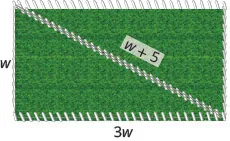154.

Nautical flags are used to represent letters of the alphabet. The flag for the letter O consists of a yellow right triangle and a red right triangle which are sewn together along their hypotenuse to form a square. The adjoining side of the two triangles is three inches longer than a side of the flag. Find the length of the side of the flag.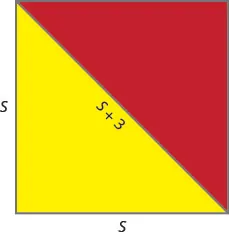155.

The length of a rectangular driveway is five feet more than three times the width. The area is 350 square feet. Find the length and width of the driveway.

156.

A rectangular lawn has area 140 square yards. Its width that is six less than twice the length. What are the length and width of the lawn?

157.

A firework rocket is shot upward at a rate of 640 ft/sec. Use the projectile formula $h=−16t2+v0th=−16t2+v0t$ to determine when the height of the firework rocket will be 1200 feet.

158.

An arrow is shot vertically upward at a rate of 220 feet per second. Use the projectile formula $h=−16t2+v0th=−16t2+v0t$ to determine when height of the arrow will be 400 feet.

#### Everyday Math

159.

A bullet is fired straight up from a BB gun with initial velocity 1120 feet per second at an initial height of 8 feet. Use the formula $h=−16t2+v0t+8h=−16t2+v0t+8$ to determine how many seconds it will take for the bullet to hit the ground. (That is, when will $h=0h=0$ ?)

160.

A city planner wants to build a bridge across a lake in a park. To find the length of the bridge, he makes a right triangle with one leg and the hypotenuse on land and the bridge as the other leg. The length of the hypotenuse is 340 feet and the leg is 160 feet. Find the length of the bridge.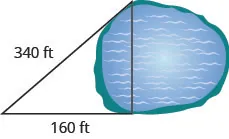#### Writing Exercises

161.

Make up a problem involving the product of two consecutive odd integers. Start by choosing two consecutive odd integers. What are your integers? What is the product of your integers? Solve the equation $n(n+2)=pn(n+2)=p$, where $pp$ is the product you found in part (b). Did you get the numbers you started with?

162.

Make up a problem involving the product of two consecutive even integers. Start by choosing two consecutive even integers. What are your integers? What is the product of your integers? Solve the equation $n(n+2)=pn(n+2)=p$, where $pp$ is the product you found in part (b). Did you get the numbers you started with?

#### Self Check

After completing the exercises, use this checklist to evaluate your mastery of the objectives of this section.On a scale of 1–10, how would you rate your mastery of this section in light of your responses on the checklist? How can you improve this?

Order a print copy

As an Amazon Associate we earn from qualifying purchases.